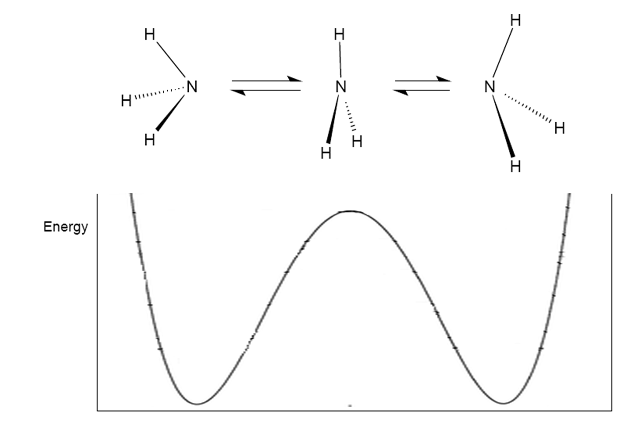#### You may also likeA quadrilateral changes shape with the edge lengths constant. Show the scalar product of the diagonals is constant. If the diagonals are perpendicular in one position are they always perpendicular?As a quadrilateral Q is deformed (keeping the edge lengths constnt) the diagonals and the angle X between them change. Prove that the area of Q is proportional to tanX.### Air Routes

Find the distance of the shortest air route at an altitude of 6000 metres between London and Cape Town given the latitudes and longitudes. A simple application of scalar products of vectors.

# Bond Angles

##### Age 16 to 18Challenge Level

Below is a diagram of the tetrahedral molecule, along with axes and labelled atoms: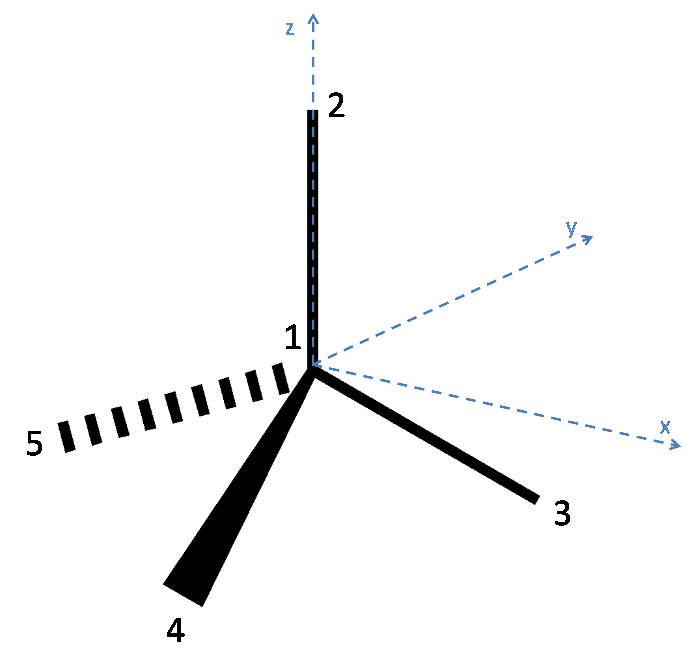As has been stated in the question, Atom 1 has coordinates (0,0,0). Additionally, since the atoms 3, 4 and 5 lie on the plane $z = -h$, it can be reasoned that Atom 2 lies on the z axis and so has coordinates (0, 0, 1).

To define the coordinates of atoms 3, 4 and 5, the x and y axes must be placed arbitrarily. To make the algebra simpler, the x axis is placed such that atom 3 lies along it. Thus the coordinates of Atom 3 are (x,0,-h) where x is a quantity to be determined. Since each bond is of unit length, x can be found as follows:

$\sqrt{x^2 + h^2} = 1$
$\therefore x = \sqrt{1 - h^2}$

Thus Atom 3 has coordinates ($\mathbf{\sqrt{1 - h^2}}$,0,-h).

To find the coordinates of atoms 4 and 5, it is easier to view the tetrahedron by looking down the z-axis: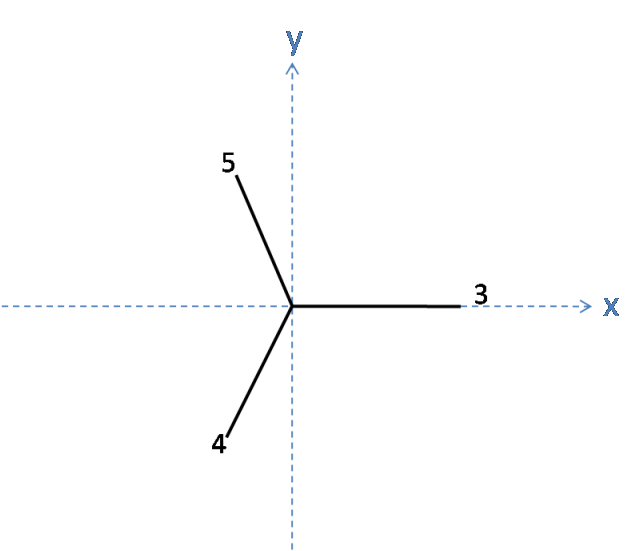From here, it can be seen that the three bonds are equally spaced and so must be separated by an angle of 120$^\circ$ in the plane. It has already been shown that the length of each of these bonds is $x$ in the plane, and so the length along the x and y axes for Atoms 4 and 5 can be easily calculated using trigonometry:

Atom 5 has $x\cos(30)$ along the y axis, and $-x\sin(30)$ along the x axis.
Atom 4 has $-x\cos(30)$ along the y axis and $-x\sin(30)$ along the x axis.

As the atoms 3, 4 and 5 lie in the plane z = -h, the coordinates for atoms 4 and 5 are as follows:

Atom 4: ( $-x\sin(30)$, $-x\cos(30)$, -h) = ($\mathbf{-\sqrt{1 - h^2}\sin(30), -\sqrt{1-h^2}\cos(30), -h}$)
Atom 5: ( $-x\sin(30)$, $x\cos(30)$, -h) = ($\mathbf{-\sqrt{1 - h^2}\sin(30), \sqrt{1-h^2}\cos(30), -h}$)

The coordinates of the atoms 2, 3, 4, and 5 have now been found. Their position vectors from Atom 1 are the same as their coordinates, since Atom 1 is at the origin. Using scalar products between pairs of position vectors, with the knowledge that their angular separation (i.e. bond angle) should be the same, yields values for h and also for the bond angle:

Dotting 2 and 3: $-h = \cos(\theta)$

Dotting 3 and 4: $\frac{3}{2}h^2 + \frac{1}{2} = \cos(\theta)$

Combinging these equations to eliminate $\cos(\theta)$ gives:

$3h^2 + 2h -1 =0$
$h = -1\ \text{or}\ h = \frac{1}{3}$

A positive value for h is clearly needed:
$\mathbf{h = \frac{1}{3}}$

$\therefore \mathbf{cos(\theta)} = -\frac{1}{3}$
$\rightarrow \mathbf{Bond\ angle = arccos(\frac{-1}{3})}$

There are many different ways to deform the perfect tetrahedron, which lead to a variety of different numbers of bond angles being preserved. It is possible to deform the tetrahedron in such a way that 4 bond angles remain at 109.5$^\circ$ and one bond angle is increased, and another concomitantly decreased.

Look at the tetrahedron illustrated below. Atom 2 can be moved towards Atom 3, which will leave the following angles preserved: Atom 4 to 5, Atom 4 to 3, Atom 3 to 5, and Atom 4 to 2. The last of these angles is only preserved if the bond length of Atom 4 remains fixed, such that Atom 4 moves towards atom two along the arc of a circle. It can clearly be seen also that the angle between Atom 2 and 3 decreases, and that the angle between 2 and 5 increases!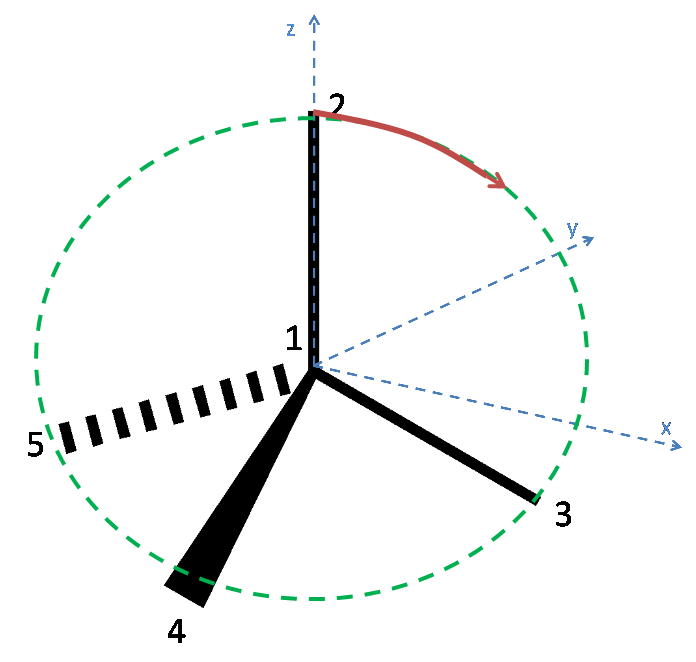The second part of this problem involves the trigonal pyramidal ammonia molecule. We have effectively moved an atom from the tetrahedron, and squashed the three remaining atoms closer together. It can be clearly visualised that the bond lengths must be increased in order to maintain the same distance between the hydrogens.To calculate what percent we must first calculate the distance between the hydrogens in the tetrahedron: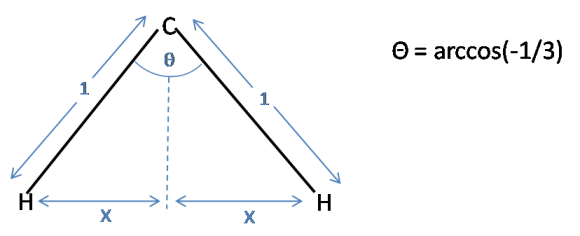Using simple trigonometry $x$ can be calculated:

$\sin(\frac{\theta}{2}) = \frac{x}{1}$
$x = \sin(\frac{1}{2}\arccos(-\frac{1}{3}))$

Now consider a triangle for the ammonia molecule. Note that the distance between the hydrogens is $2x$, as in the tetrahedron: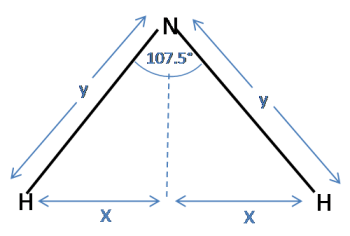Again, using trigonometry:

$\sin(\frac{107.5}{2}) = \frac{x}{y}$

Substituting for $x$ and rearranging to find $y$ gives:

$y = \frac{\sin(\frac{1}{2}\arccos(-\frac{1}{3}))}{\sin(\frac{107.5}{2})} = 1.0125$

Therefore, the percentage increase is 1.25%.

If the hydrogen atoms remained fixed and a vertical force was applied to the N atom, the maximum bond angle would be seen when the molecule became planar. In these circumstances, the three hydrogen atoms would be in the same plane as the nitrogen atom, and would be distributed around it at 120$^\circ$ intervals. Thus, the maximum theoretical bond angle for the trigonal pyramidal arrangement is $\mathbf{120^\circ}$.

Although it can be seen that the formation of the planar ammonia molecule requires much energy, this is an observed phonomenon. At room temperature, ammonia rapidly inverts (~ 10$^{12}$ times per second), much like an umbrella turning inside out in the wind. In order to invert, the molecule must pass through a high-energy unstable planar transition state: